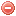# Description

The Initial spread index ($$ISI$$) is one of the two intermediate indices required for calculating the Canadian fire weather index ($$FWI$$). The $$ISI$$ represents the rate of fire spread without the influence of variable quantities of fuel. It is a combination of the effects of wind speed and fine fuel moisture content on fire spread, and thus requires wind speed and the $$FFMC$$ as input variables (Van Wagner 1987).

The name of this index refers to the basic rate at which a fire would spread when the fine fuel is dry but drying in depth not advanced (Van Wagner 1987).

As the $$ISI$$ combines the influences of wind and fine fuel moisture, it is well appropriated for predicting burnt area (Van Wagner 1987).

# Formula

The $$ISI$$ is the product of a wind and a fine fuel moisture functions.

The wind function is obtained as follows (Van Wagner and Pickett 1985):

$f(U)=e^{0.05039\cdot U_{12}}$

where $$U_{12}$$ is wind speed [km/h] at noon.

And the fine fuel moisture function as follows (Van Wagner 1985):

$f(F)=(91.9\cdot {e^{-01386\cdot{m}}})\cdot{\bigg(1+\dfrac{m^{5.31}}{4.93\cdot{10^7}}\bigg)}$

where $$m$$ is the fuel moisture content [%] and dermined as follows (Van Wagner 1985):

$m=147.2\cdot\dfrac{101-FFMC}{59.5+FFMC}$

where $$FFMC$$ is the Fine fuel moisture code.

The $$ISI$$ is finally obtained with the following equation (Van Wagner 1985):

$ISI=0.208\cdot{f(U)}\cdot{f(F)}$

Original publications:
Van Wagner and Pickett (1985)
Van Wagner (1987)

### Bibliography SearchNo results!

No results were found. Are you sure you searched for a tag?

### Symbols

 Variable Description Unit $$T$$ air temperature °C $$T_{dew}$$ dew point temperature °C $$H$$ air humidity % $$P$$ rainfall mm $$U$$ windspeed m/s $$w$$ days since last rain (or rain above threshold) d $$rr$$ days with consecutive rain d $$\Delta t$$ time increment d $$\Delta{e}$$ vapor pressure deficit kPa $$e_s$$ saturation vapor pressure kPa $$e_a$$ actual vapor pressure kPa $$p_{atm}$$ atmospheric pressure kPa $$PET$$ potential evapotranspiration mm/d $$r$$ soil water reserve mm $$r_s$$ surface water reserve mm $$EMC$$ equilibrium moisture content % $$DF$$ drought factor - $$N$$ daylight hours hr $$D$$ weighted 24-hr average moisture condition hr $$\omega$$ sunset hour angle rad $$\delta$$ solar declination rad $$\varphi$$ latitude rad $$Cc$$ cloud cover Okta $$J$$ day of the year (1..365/366) - $$I$$ heat index - $$R_n$$ net radiation MJ⋅m-2⋅d-1 $$R_a$$ daily extraterrestrial radiation MJ⋅m-2⋅d-1 $$R_s$$ solar radiation MJ⋅m-2⋅d-1 $$R_{so}$$ clear-sky solar radiation MJ⋅m-2⋅d-1 $$R_{ns}$$ net shortwave radiation MJ⋅m-2⋅d-1 $$R_{nl}$$ net longwave radiation MJ⋅m-2⋅d-1 $$\lambda$$ latent heat of vaporization MJ/kg $$z$$ elevation m a.s.l. $$d_r$$ inverse relative distance Earth-Sun - $$\alpha$$ albedo or canopy reflection coefficient - $$\Delta$$ slope of the saturation vapor pressure curve kPa/°C $$Cc$$ cloud cover eights $$ROS$$ rate of spread m/h $$RSF$$ rate of spread factor - $$WF$$ wind factor - $$WRF$$ water reserve factor - $$FH$$ false relative humidity - $$FAF$$ fuel availability factor - $$PC$$ phenological coefficient -

 Suffix Description $$-$$ mean / daily value $$_{max}$$ maximum value $$_{min}$$ minimum value $$_{12}$$ value at 12:00 $$_{13}$$ value at 13:00 $$_{15}$$ value at 15:00 $$_{m}$$ montly value $$_{y}$$ yearly value $$_{f/a}$$ value at fuel-atmosphere interface $$_{dur}$$ duration $$_{soil}$$ value at soil level

 Constant Description $$e$$ Euler's number $$\gamma$$ psychrometric constant $$G_{SC}$$ solar constant $$\sigma$$ Stefan-Bolzmann constant